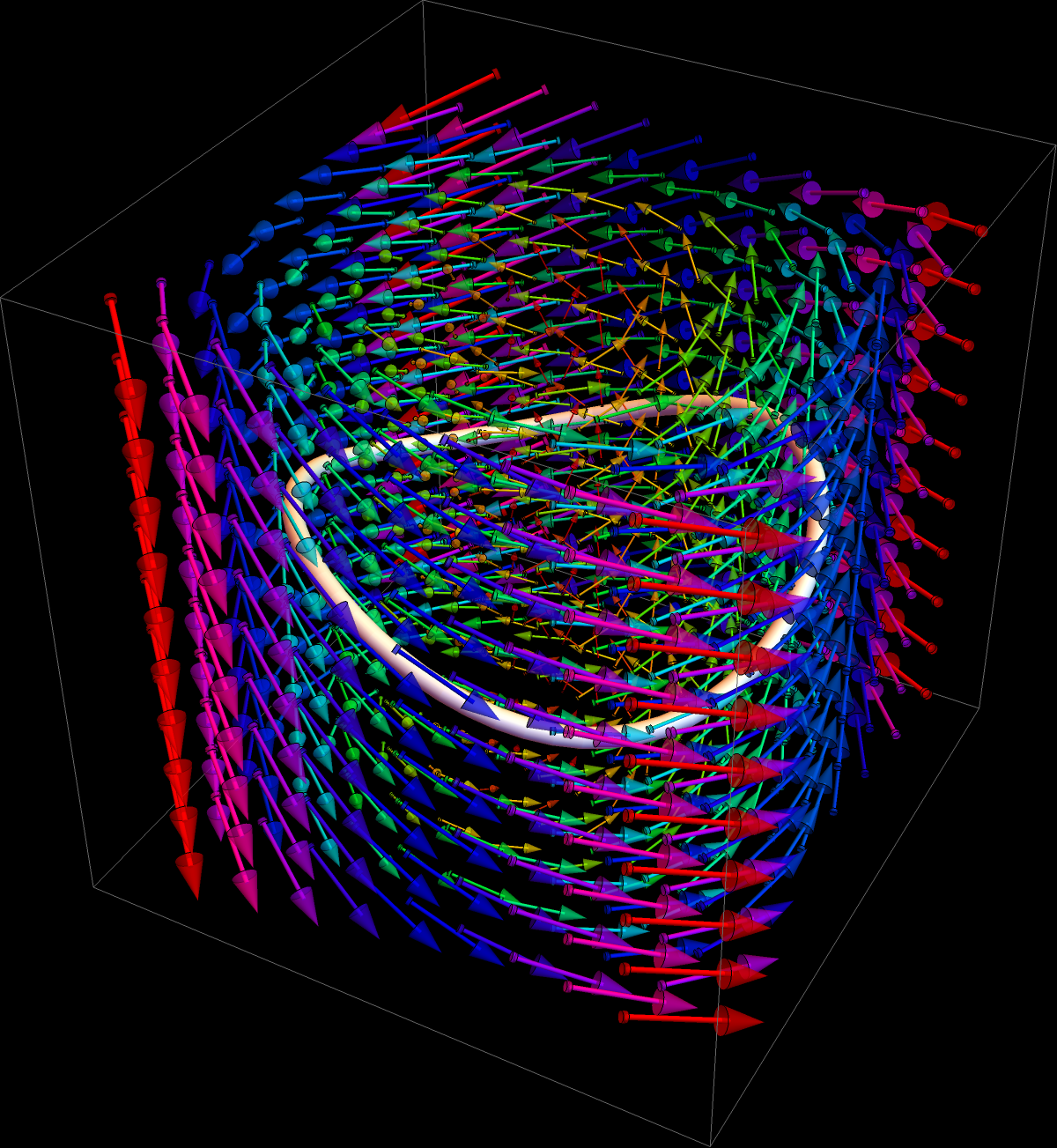## Can one force curl(F) to be perpendicular to F?

In the final exam of 2014 seen here we have asked whether it is true that the curl of a vector field F is always perpendicular to F. It is not as one can add a general constant field to a given field without changing the curl. One more generally can add a gradient field to F and still get the same curl.
One is seduced to think that curl(F).F = 0 because Nabla calculus tells that curl(F) = ∇ x F is a cross product of ∇ with F, and because we know that the cross product of two vector is perpendicular to both.
A counter example is F = ⟨ 0,x,1 ⟩ for which the curl is ⟨ 0,0,1 ⟩ After a student insisted on knowing more about the reason why it fails, I mentioned in the answer to the student that the following might be true but that I would not know whether it is true:

 Given a vector field F=⟨ P,Q,R ⟩. Can one add a gradient field ∇ f so that F + ⟨ fx,fy,fz ⟩ is perpendicular to F?

In other words, is it true that modulo gradient fields, the orthogonality condition is true? I (Oliver) thought that the answer is yes, as for a given F, this is a simple partial differential equation for an unknown function f. The PDE is

 ``` curl(F). F = curl(F) . ∇ f ```

so that it is likely that one of the existence theorems for solutions of PDEs applies. I asked around in our group. It was Jake Marcinek who came up with a solution.
Jake found that the answer is "no", if the curl(F) has a closed loop flow line.

First of all, Jake thinks about curl(F) . ∇ f as a directional derivative of f in the direction curl(F). If curl(F) has a closed flow line, curl(F) (r(t)). r'(t) is positive for all t so that the directional derivatives are all positive. But this is not possible as this would mean f always increases along the curve but returns to the same point.
A concrete example given by Jake is

 ```F=⟨ -2y+4y3/3,0,-(x2 + y2)/2 ⟩ ```

for which

 ```curl(F) = ⟨ -y,x,2-4y2 ⟩ ```

has the closed flow line r(t) = ⟨ cos(t),sin(t),sin(2t) ⟩. But F.curl(F) = y2 + 2 y4/3 + 2x2 y2 - x2 integrates positively over any closed loop that projects onto the unit circle.

If you want to know more, ask Jake. He has seen more connections like to quaternions and contact forms. It all indicates that this global existence problem is more interesting than anticipated.
Here is the vector field curl(F) in Jake's example